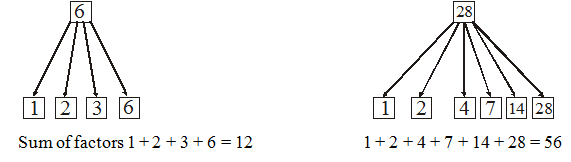# Introduction

## Playing With Numbers of Class 6

### Introduction

A number can be expressed as the product of different numbers as

6 = 1 × 6

6 = 2 × 3

12 = 1 × 12

12 = 3 × 4

12 = 2 × 6

Also 6 is exactly divisible by 1, 2, 3 and 6. Similarly 12 is exactly divisible by 1, 2, 3, 4, 6 and 12. Hence 1, 2, 3 and 6 are factors of 6 and 1, 2, 3,4, 6 and 12 are factors of 12.

### Factors

A factor of a number is an exact divisor of that number.

Illustration 1:

Find the factor of 24, 20 and 48.

Sol.

24 = 1 × 24

24 = 2 × 12

24 = 4 × 6

24 = 3 × 8

Factors are 1, 2, 3, 4, 6, 8, 12 and 24

20 = 1 × 20

20 = 2 × 10

20 = 4 × 5

Factors are 1, 2, 4, 5, 10 and 20.

48 = 1 × 48

48 = 2 × 24

48 = 3 × 16

48 = 4 × 12

48 = 6 × 8

Factors are 1, 2, 3, 4, 6, 8, 12, 16, 24 and 48.The number whose sum of the factors is double of that number are called perfect number.

Multiples

1 × 24 = 24

2 × 12 = 24

3 × 8 = 24

4 × 6 = 24

1, 2, 3, 4, 6, 8 and 12, 24 are factors of 24

But 24 is multiple of its factors.

Now look at this

2, 4, 6, 8, …….

3, 6, 9, 12, …….

Here each number is multiple of preceding number. Every succeeding number is divisible by preceding number.

Definition

Each number is multiple of its factor.

Note: Multiple of any number can be obtained by multiplying the number with any number.

Prime numbers

Those numbers which have only two factors 1 and itself are called prime numbers.

Composite numbers

All numbers other than prime numbers are called composite numbers. Composite numbers have more than two factors.

Illustration 2:

Seperate prime and composite numbers from the following group.

2, 5, 6, 7, 17, 19, 20, 23, 33, 35, 39, 41

Sol. Prime: 2, 5, 7, 17, 19, 23, 41

Composite : 6, 20, 33, 35, 39

Note: 1 is neither prime nor composite number.

Note: 2 is the smallest prime number.

### Even and odd numbers

All the multiples of 2 are called even while all the numbers which are not multiples of 2, are called odd numbers.

e.g., 2, 4, 6, 8, 10……. are even numbers.

1, 3, 5, 7, 9, 11 ……. are odd numbers.